Question

The drawing shows a collision between two pucks on an air-hockey table. Puck A has a mass of 0.26 kg and is moving along the x axis with a velocity of 5.60 m/s. It makes a collision with puck B, which has a mass of 0.52 kg and is initially at rest. After the collision, the two pucks fly apart with angles as shown in the drawing (α = 56° and β = 40°). Find the final speed of puck B.Note that, you did not provide the image

#### Earn Coins

Coins can be redeemed for fabulous gifts.

Similar Homework Help Questions
• ### The drawing shows a collision between two pucks on an air-hockey table. Puck A has a...

The drawing shows a collision between two pucks on an air-hockey table. Puck A has a mass of 0.28 kg and is moving along the x axis with a velocity of 5.80 m/s. It makes a collision with puck B, which has a mass of 0.56 kg and is initially at rest. After the collision, the two pucks fly apart with angles as shown in the drawing (α = 56° and β = 40°). Find the final speed of puck...

• ### The drawing shows a collision between two pucks on an air-hockey table. Puck A has a...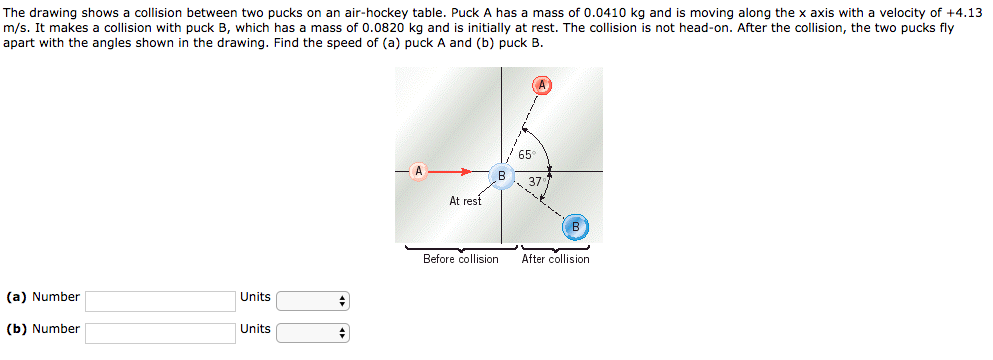The drawing shows a collision between two pucks on an air-hockey table. Puck A has a mass of 0.0410 kg and is moving along the x axis with a velocity of +4.13 m/s. It makes a collision with puck B, which has a mass of 0.0820 kg and is initially at rest. The collision is not head-on. After the collision, the two pucks fly apart with the angles shown in the drawing. Find the speed of (a) puck A and...

• ### The drawing shows a collision between two pucks on an air-hockey table. Puck A has a...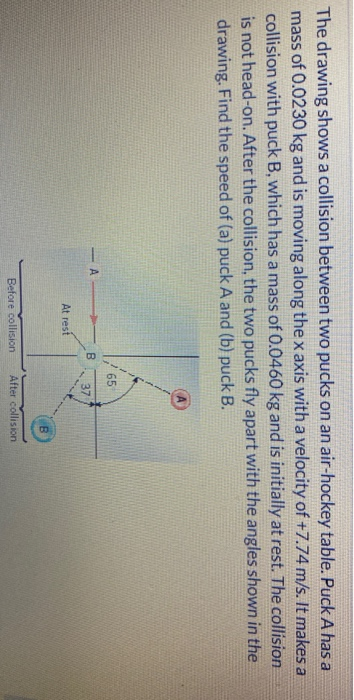The drawing shows a collision between two pucks on an air-hockey table. Puck A has a mass of 0.0230 kg and is moving along the x axis with a velocity of +7.74 m/s. It makes a collision with puck B, which has a mass of 0.0460 kg and is initially at rest. The collision is not head-on. After the collision, the two pucks fly apart with the angles shown in the drawing. Find the speed of (a) puck A and...

• ### The drawing shows a collision between two pucks on an air-hockey table. Puck A has a...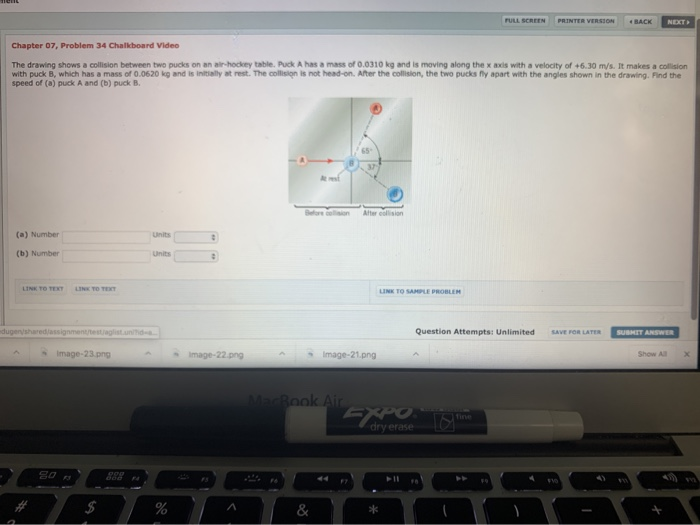The drawing shows a collision between two pucks on an air-hockey table. Puck A has a mass of 0.0310 kg and is moving along the x axis with a velocity of +6.30 m/s. It makes a collison with Puck B, which had a mass of 0.0620 kg and is initially at rest. The collision is not head-on. After the collision, the two pucks fly apart woth the angles shown in the drawing. Find the speed of (a) puck A and...

• ### 6. The drawing shows a collision between two pucks on an air-hockey table. Puck A has...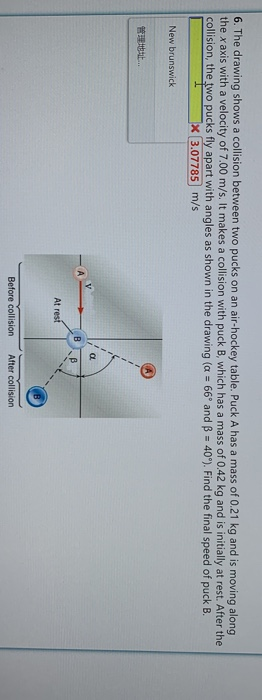6. The drawing shows a collision between two pucks on an air-hockey table. Puck A has a mass of 0.21 kg and is moving along the x axis with a velocity of 7.00 m/s. It makes a collision with puck B, which has a mass of 0.42 kg and is initially at rest. After the collision, the two pucks fly apart with angles as shown in the drawing (a 66° and B 40°). Find the final speed of puck B....

• ### Chapter 07, Problem 34 Chalkboard Video The drawing shows a collision between two pucks on an...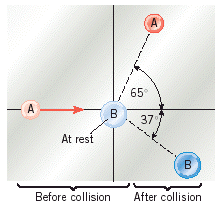Chapter 07, Problem 34 Chalkboard Video The drawing shows a collision between two pucks on an air-hockey table. Puck A has a mass of 0.0170 kg and is moving along the x axis with a velocity of +4.65 m/s. It makes a collision with puck B, which has a mass of 0.0340 kg and is initially at rest. The collision is not head-on. After the collision, the two pucks fly apart with the angles shown in the drawing . Find...

• ### Multiple concepts

Multiple-Concept Example 9 reviews the concepts that play roles in this problem. The drawing shows a collision between two pucks on an air-hockey table. Puck A has amass of 0.0190 kg and is moving along the x axis with a velocity of +7.31 m/s. It makes a collision with puck B, which has a mass of 0.0380 kg and is initially atrest. The collision is not head-on. After the collision, the two pucks fly apart with the angles shown in...

• ### Two pucks collide on an air hockey table. Puck A has a mass of 17.0 g...

Two pucks collide on an air hockey table. Puck A has a mass of 17.0 g and is initially traveling in the +x direction at 7.80 m/s. Puck B has a mass of 68.0 g and is initially at rest. After the pucks collide, puck A moves away at an angle of 58.0 above the +x axis, while puck B travels at an angle of 24.0 below the +x axis. Calculate puck A's final speed? Calculate puck B's final speed?...

• ### Two pucks collide on an air hockey table. Puck A has a mass of 28 g...

Two pucks collide on an air hockey table. Puck A has a mass of 28 g and is initially traveling in the +x direction at 7.8 m/s. Puck B has a mass of 112 g and is initially at rest. After the pucks collide, puck A moves away at an angle of 45\$^\circ\$ above the +x axis, while puck B travels at an angle of 42\$^\circ\$ below the +x axis. Calculate puck A's final speed and puck B's final speed....

• ### wo pucks collide on an air hockey table. Puck A has a mass of 26.0 g...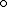wo pucks collide on an air hockey table. Puck A has a mass of 26.0 g and is initially traveling in the +xdirection at 6.50 m/s. Puck B has a mass of 78.0 g and is initially at rest. After the pucks collide, puck A moves away at an angle of 58.0 above the +x axis, while puck B travels at an angle of 26.0 below the +x axis. Calculate puck A's final speed. Calculate puck B's final speed. What...